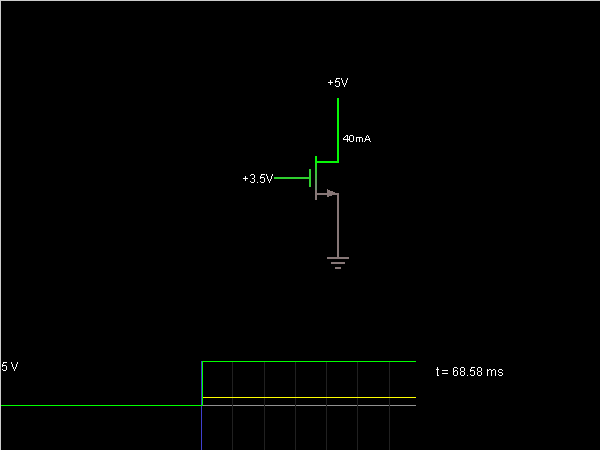# Circuit Simulator - n-MOSFET

n-MOSFETCircuit Description:
This is a simple model of a n-type MOSFET. The source is at ground, and the gate and drain voltages can be controlled using the sliders at the right.

Basically no current flows if the gate voltage is below the threshold voltage (1.5 V). When you raise it above that, current begins to flow.

When the gate and drain voltages are sufficiently high, the MOSFET is in saturation, and the current is constant regardless of the drain voltage. If you lower the drain voltage enough relative to the gate, then the MOSFET is in linear mode, and the current is roughly linear to the drain voltage. By sliding the drain voltage slider slowly back and forth, you should be able to see the boundary between the linear and saturation regions. Try this with different settings for the gate voltage.

Discussion:
Be the first person to comment on this question !